Open in App
Not now

# Postorder Traversal of Binary Tree

• Last Updated : 23 Mar, 2023

Postorder traversal is defined as a type of tree traversal which follows the Left-Right-Root policy such that for each node:

• The left subtree is traversed first
• Then the right subtree is traversed
• Finally, the root node of the subtree is traversed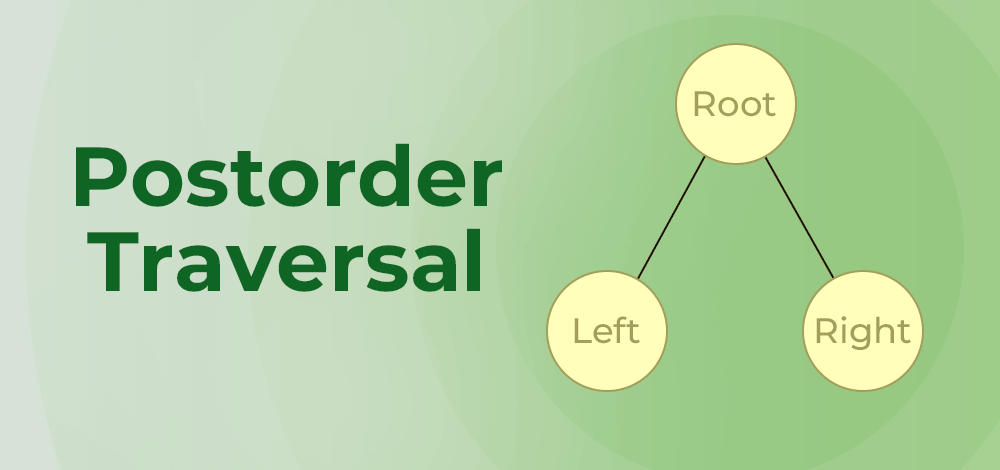Postorder traversal

## Algorithm for Postorder Traversal of Binary Tree:

The algorithm for postorder traversal is shown as follows:

Postorder(root):

1. Follow step 2 to 4 until root != NULL
2. Postorder (root -> left)
3. Postorder (root -> right)
4. Write root -> data
5. End loop

## How does Postorder Traversal of Binary Tree Work?

Consider the following tree: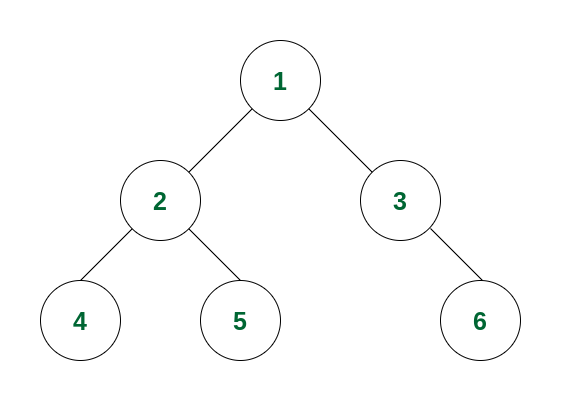Example of Binary Tree

If we perform a postorder traversal in this binary tree, then the traversal will be as follows:

Step 1: The traversal will go from 1 to its left subtree i.e., 2, then from 2 to its left subtree root, i.e., 4. Now 4 has no subtree, so it will be visited.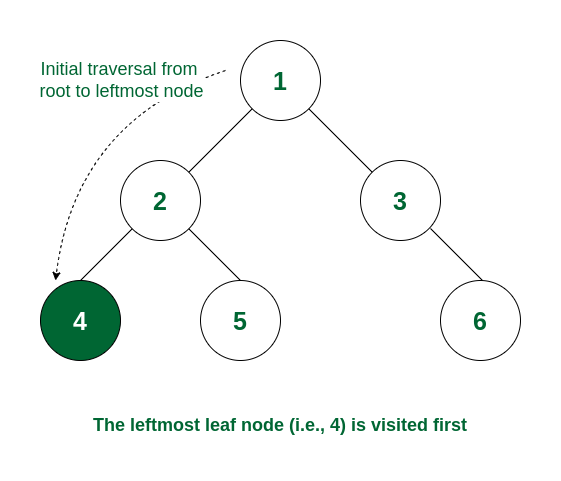Node 4 is visited

Step 2: As the left subtree of 2 is visited completely, now it will traverse the right subtree of 2 i.e., it will move to 5. As there is no subtree of 5, it will be visited.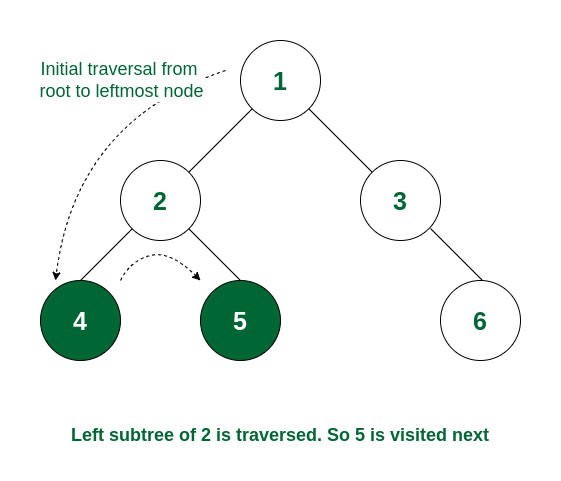Node 5 is visited

Step 3: Now both the left and right subtrees of node 2 are visited. So now visit node 2 itself.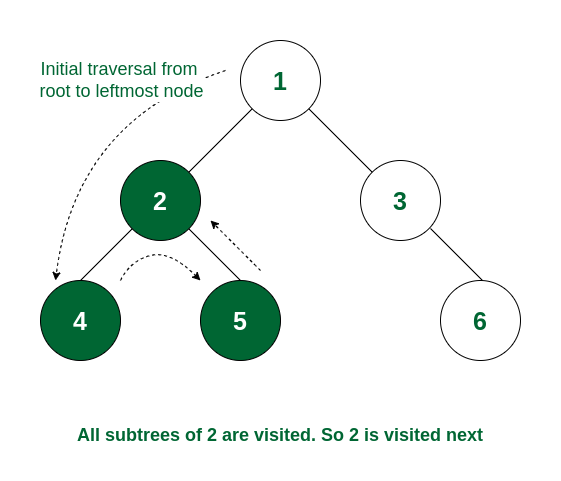Node 2 is visited

Step 4: As the left subtree of node 1 is traversed, it will now move to the right subtree root, i.e., 3. Node 3 does not have any left subtree, so it will traverse the right subtree i.e., 6. Node 6 has no subtree and so it is visited.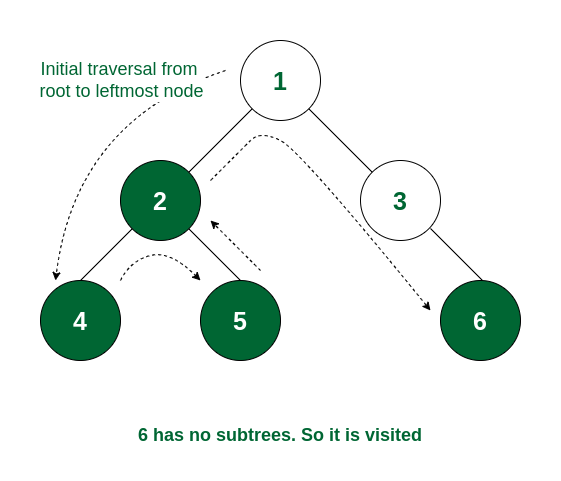Node 6 is visited

Step 5: All the subtrees of node 3 are traversed. So now node 3 is visited.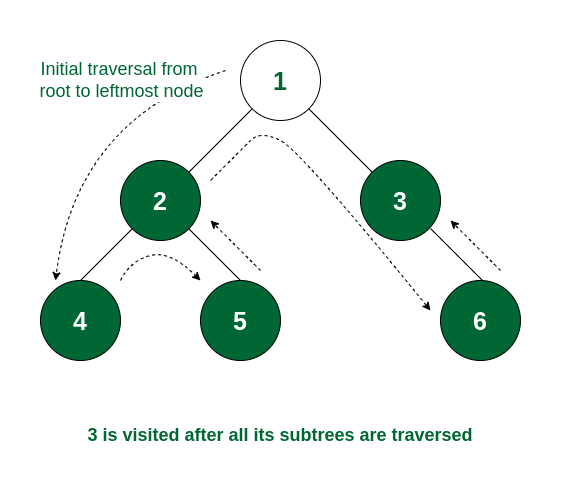Node 3 is visited

Step 6: As all the subtrees of node 1 are traversed, now it is time for node 1 to be visited and the traversal ends after that as the whole tree is traversed.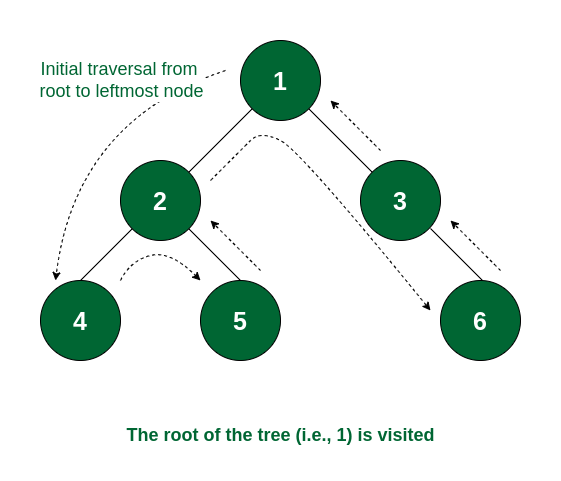The complete tree is visited

So the order of traversal of nodes is 4 -> 5 -> 2 -> 6 -> 3 -> 1.

## Program to implement Postorder Traversal of Binary Tree

Below is the code implementation of the postorder traversal:

## C++

 `// C++ program for postorder traversals`   `#include ` `using` `namespace` `std;`   `// Structure of a Binary Tree Node` `struct` `Node {` `    ``int` `data;` `    ``struct` `Node *left, *right;` `    ``Node(``int` `v)` `    ``{` `        ``data = v;` `        ``left = right = NULL;` `    ``}` `};`   `// Function to print postorder traversal` `void` `printPostorder(``struct` `Node* node)` `{` `    ``if` `(node == NULL)` `        ``return``;`   `    ``// First recur on left subtree` `    ``printPostorder(node->left);`   `    ``// Then recur on right subtree` `    ``printPostorder(node->right);`   `    ``// Now deal with the node` `    ``cout << node->data << ``" "``;` `}`   `// Driver code` `int` `main()` `{` `    ``struct` `Node* root = ``new` `Node(1);` `    ``root->left = ``new` `Node(2);` `    ``root->right = ``new` `Node(3);` `    ``root->left->left = ``new` `Node(4);` `    ``root->left->right = ``new` `Node(5);` `    ``root->right->right = ``new` `Node(6);`   `    ``// Function call` `    ``cout << ``"Postorder traversal of binary tree is: \n"``;` `    ``printPostorder(root);`   `    ``return` `0;` `}`

## Java

 `// Java program for postorder traversals` `import` `java.util.*;`   `// Structure of a Binary Tree Node` `class` `Node {` `    ``int` `data;` `    ``Node left, right;` `    ``Node(``int` `v)` `    ``{` `        ``data = v;` `        ``left = right = ``null``;` `    ``}` `}`   `class` `GFG {` `    `  `    ``// Function to print postorder traversal` `    ``static` `void` `printPostorder(Node node)` `    ``{` `        ``if` `(node == ``null``)` `            ``return``;`   `        ``// First recur on left subtree` `        ``printPostorder(node.left);`   `        ``// Then recur on right subtree` `        ``printPostorder(node.right);`   `        ``// Now deal with the node` `        ``System.out.print(node.data + ``" "``);` `    ``}`   `    ``// Driver code` `    ``public` `static` `void` `main(String[] args)` `    ``{` `        ``Node root = ``new` `Node(``1``);` `        ``root.left = ``new` `Node(``2``);` `        ``root.right = ``new` `Node(``3``);` `        ``root.left.left = ``new` `Node(``4``);` `        ``root.left.right = ``new` `Node(``5``);` `        ``root.right.right = ``new` `Node(``6``);`   `        ``// Function call` `        ``System.out.println(``"Postorder traversal of binary tree is: "``);` `        ``printPostorder(root);` `    ``}` `}` `// This code is contributed by prasad264`

## Python3

 `# Python program for postorder traversals`   `# Structure of a Binary Tree Node`     `class` `Node:` `    ``def` `__init__(``self``, v):` `        ``self``.data ``=` `v` `        ``self``.left ``=` `None` `        ``self``.right ``=` `None`   `# Function to print postorder traversal`     `def` `printPostorder(node):` `    ``if` `node ``=``=` `None``:` `        ``return`   `    ``# First recur on left subtree` `    ``printPostorder(node.left)`   `    ``# Then recur on right subtree` `    ``printPostorder(node.right)`   `    ``# Now deal with the node` `    ``print``(node.data, end``=``' '``)`     `# Driver code` `if` `__name__ ``=``=` `'__main__'``:` `    ``root ``=` `Node(``1``)` `    ``root.left ``=` `Node(``2``)` `    ``root.right ``=` `Node(``3``)` `    ``root.left.left ``=` `Node(``4``)` `    ``root.left.right ``=` `Node(``5``)` `    ``root.right.right ``=` `Node(``6``)`   `    ``# Function call` `    ``print``(``"Postorder traversal of binary tree is:"``)` `    ``printPostorder(root)`

## C#

 `// C# program for postorder traversals`   `using` `System;`   `// Structure of a Binary Tree Node` `public` `class` `Node {` `    ``public` `int` `data;` `    ``public` `Node left, right;` `    ``public` `Node(``int` `v)` `    ``{` `        ``data = v;` `        ``left = right = ``null``;` `    ``}` `}`   `public` `class` `GFG {`   `    ``// Function to print postorder traversal` `    ``static` `void` `printPostorder(Node node)` `    ``{` `        ``if` `(node == ``null``)` `            ``return``;`   `        ``// First recur on left subtree` `        ``printPostorder(node.left);`   `        ``// Then recur on right subtree` `        ``printPostorder(node.right);`   `        ``// Now deal with the node` `        ``Console.Write(node.data + ``" "``);` `    ``}`   `    ``static` `public` `void` `Main()` `    ``{`   `        ``// Code` `        ``Node root = ``new` `Node(1);` `        ``root.left = ``new` `Node(2);` `        ``root.right = ``new` `Node(3);` `        ``root.left.left = ``new` `Node(4);` `        ``root.left.right = ``new` `Node(5);` `        ``root.right.right = ``new` `Node(6);`   `        ``// Function call` `        ``Console.WriteLine(` `            ``"Postorder traversal of binary tree is: "``);` `        ``printPostorder(root);` `    ``}` `}`   `// This code is contributed by karthik.`

## Javascript

 `// Structure of a Binary Tree Node` `class Node {` `  ``constructor(v) {` `    ``this``.data = v;` `    ``this``.left = ``null``;` `    ``this``.right = ``null``;` `  ``}` `}`   `// Function to print postorder traversal` `function` `printPostorder(node) {` `  ``if` `(node == ``null``) {` `    ``return``;` `  ``}`   `  ``// First recur on left subtree` `  ``printPostorder(node.left);`   `  ``// Then recur on right subtree` `  ``printPostorder(node.right);`   `  ``// Now deal with the node` `  ``console.log(node.data + ``" "``);` `}`   `// Driver code` `function` `main() {` `  ``let root = ``new` `Node(1);` `  ``root.left = ``new` `Node(2);` `  ``root.right = ``new` `Node(3);` `  ``root.left.left = ``new` `Node(4);` `  ``root.left.right = ``new` `Node(5);` `  ``root.right.right = ``new` `Node(6);`   `  ``// Function call` `  ``console.log(``"Postorder traversal of binary tree is: \n"``);` `  ``printPostorder(root);` `}`   `main();`

Output

```Postorder traversal of binary tree is:
4 5 2 6 3 1 ```

Explanation: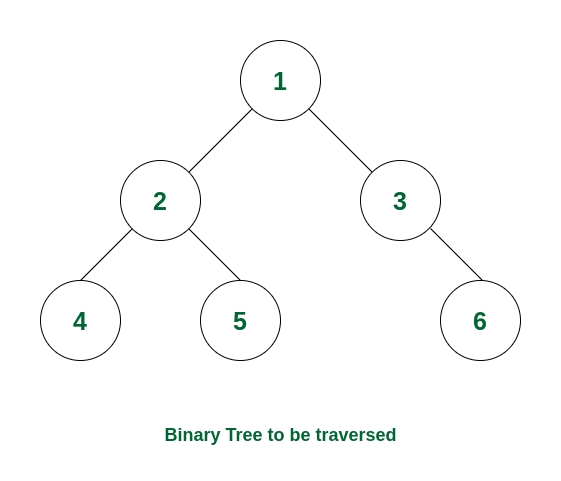How postorder traversal works

### Complexity Analysis:

Time Complexity: O(N) where N is the total number of nodes. Because it traverses all the nodes at least once.
Auxiliary Space: O(1) if no recursion stack space is considered. Otherwise, O(h) where h is the height of the tree

• In the worst case, h can be the same as N (when the tree is a skewed tree)
• In the best case, h can be the same as logN (when the tree is a complete tree)

### Use cases of Postorder Traversal:

Some use cases of postorder traversal are:

• This is used for tree deletion.
• It is also useful to get the postfix expression from an expression tree.

Related articles:

My Personal Notes arrow_drop_up
Related Articles